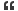1. ## simple math question

hi friends ,

If the difference of two numbers is 8 and their product is 12, what is the sum of their squares?Reply With Quote

2. ## Re: About cpp connectivity

There is no possible answer for this...You told their product is 12. So the possible combination is (1,12),(2,6),(3,4)

Whether the number is +ve or -ve, there is no possible for getting the difference 8.

If i am wrong then correct me...otherwise correct your question...

--------------------
sureshReply With Quote

3. ## Re: About cpp connectivityOriginally Posted by psuresh1982There is no possible answer for this...You told their product is 12. So the possible combination is (1,12),(2,6),(3,4)

Whether the number is +ve or -ve, there is no possible for getting the difference 8.

If i am wrong then correct me...otherwise correct your question...

--------------------
suresh

hi suresh .

i was given that question by an interview . i also confused with question . still i don't know correct answer . As u told question may be wrong i accept it ..Reply With Quote

4. ## Re: About cpp connectivity

It is given that a -b = 8
and ab = 12

we need to calculate a^2 + b^2

We know that a^2 + b^2 = (a-b)^2 + 2ab
= 8^2 + 2 *12
= 88

To Suresh, It is not given that both a and b are integers and you are not asked to find the values of a and b.

Hope this helps you guys.Reply With Quote

5. ## Re: About cpp connectivity

hi james,

Nice explanation. Now i am clear about the question....

----------------
sureshReply With Quote

6. ## Re: About cpp connectivity

that was really tricky, so is the answer.Reply With Quote

####Posting Permissions

• You may not post new threads
• You may not post replies
• You may not post attachments
• You may not edit your posts
•